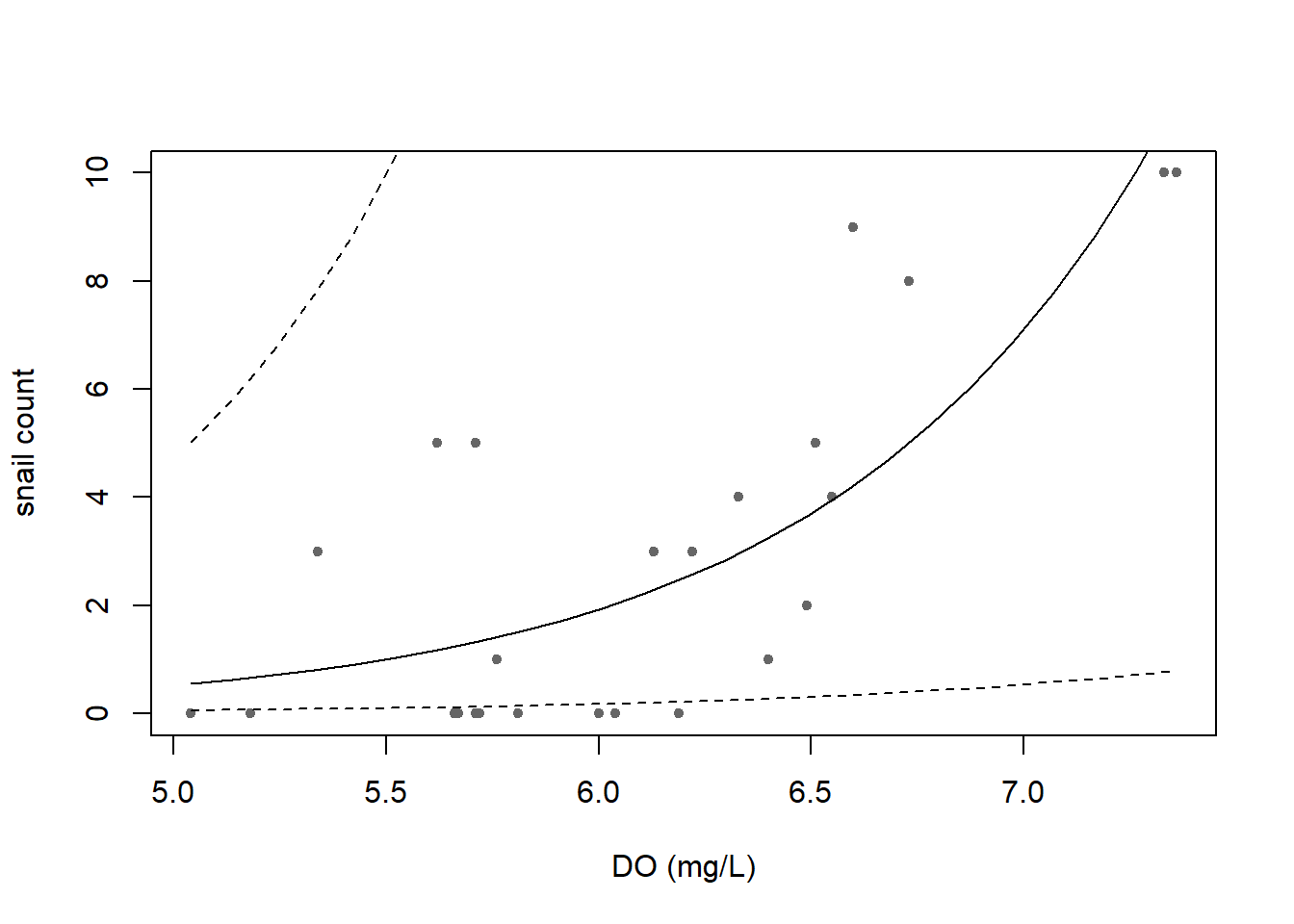## R Markdown

This is an R Markdown document. Markdown is a simple formatting syntax for authoring HTML, PDF, and MS Word documents. For more details on using R Markdown see http://rmarkdown.rstudio.com.

When you click the Knit button a document will be generated that includes both content as well as the output of any embedded R code chunks within the document. You can embed an R code chunk like this:

print("Hello world!")
##  "Hello world!"

Use the green play button in the top right corner of the code chunk to run the code (or all the code in the file). For this lesson, let’s run each chunk one at a time.

Ok, lets get started. You’ve downloaded the datasheet right? It’s a .csv file called “EnoRiverData.csv”. Do you remember where you put it? Maybe it’s in a folder on your Desktop? Great, lets set the working directory to that location and the read in the data file.

Look in the upper right panel, under the Environment tab…do you see the data file there. Good. If not, check to make sure that this markdown file is saved in the same location as the data file and that also matches the working directory (setwd).

Let’s inspect the data:

head(data)
##   X Site Meters.Downstream Position DO_mgL DO_pctsat temperature_C SpCond

## 1 1    1                 1        3   6.51      85.2          29.0  145.6

## 2 2    2                 7        2   5.81      76.8          29.0  146.1

## 3 3    3                12        1   6.49      83.6          28.6  145.6

## 4 4    4                16        5   5.62      74.7          29.9  179.2

## 5 5    5                30        5   7.33     100.0          31.1  178.6

## 6 6    6                37        4   6.60      86.6          29.0  145.5

##     pH Depth_m Velocity snail_count Total_pct_plant.algae_cover

## 1 7.50    0.48     0.30           5                          73

## 2 7.49    0.03     0.04           0                           1

## 3 7.51    0.16     0.20           2                           2

## 4 7.73    0.14     0.01           5                           0

## 5 8.01    0.08     0.01          10                           0

## 6 7.69    0.13     0.65           9                          90

##   pct_coverBelowSurface pct_coverAboveSurface

## 1                   100                     0

## 2                   100                     0

## 3                   100                     0

## 4                     0                     0

## 5                     0                     0

## 6                    97                     3

##                                                        AutotrophCrewNotes

## 1                                                           all species 1

## 2                                                               species 1

## 3                                                          100% species 2

## 4                                                            all sediment

## 5

## 6 some above, most fallen - 6 individuals of species 4, most of species 5

Ok, looks like stream data. I see sites and some other stuff. Ok, there’s pH, we know what that is. Snail count! Nice. Let’s do something with that:

# How many sites are in the dataset

nrow(data)
##  25
# Get the summary statistics for snail count

summary(data$snail_count) ## Min. 1st Qu. Median Mean 3rd Qu. Max. ## 0.00 0.00 2.00 2.92 5.00 10.00 # What was the snail count at site #5 data$snail_count[which(data$Site == 5)] ##  10 We can also subset the data: #Let's look at only the really shallow sites data[which(data$Depth_m < 0.10), 1:6]
##     X Site Meters.Downstream Position DO_mgL DO_pctsat

## 2   2    2                 7        2   5.81      76.8

## 5   5    5                30        5   7.33     100.0

## 15 15   15               114        1   5.18      65.1

How about we see the entire distribution of depts with a histogram?

hist(data$Depth_m)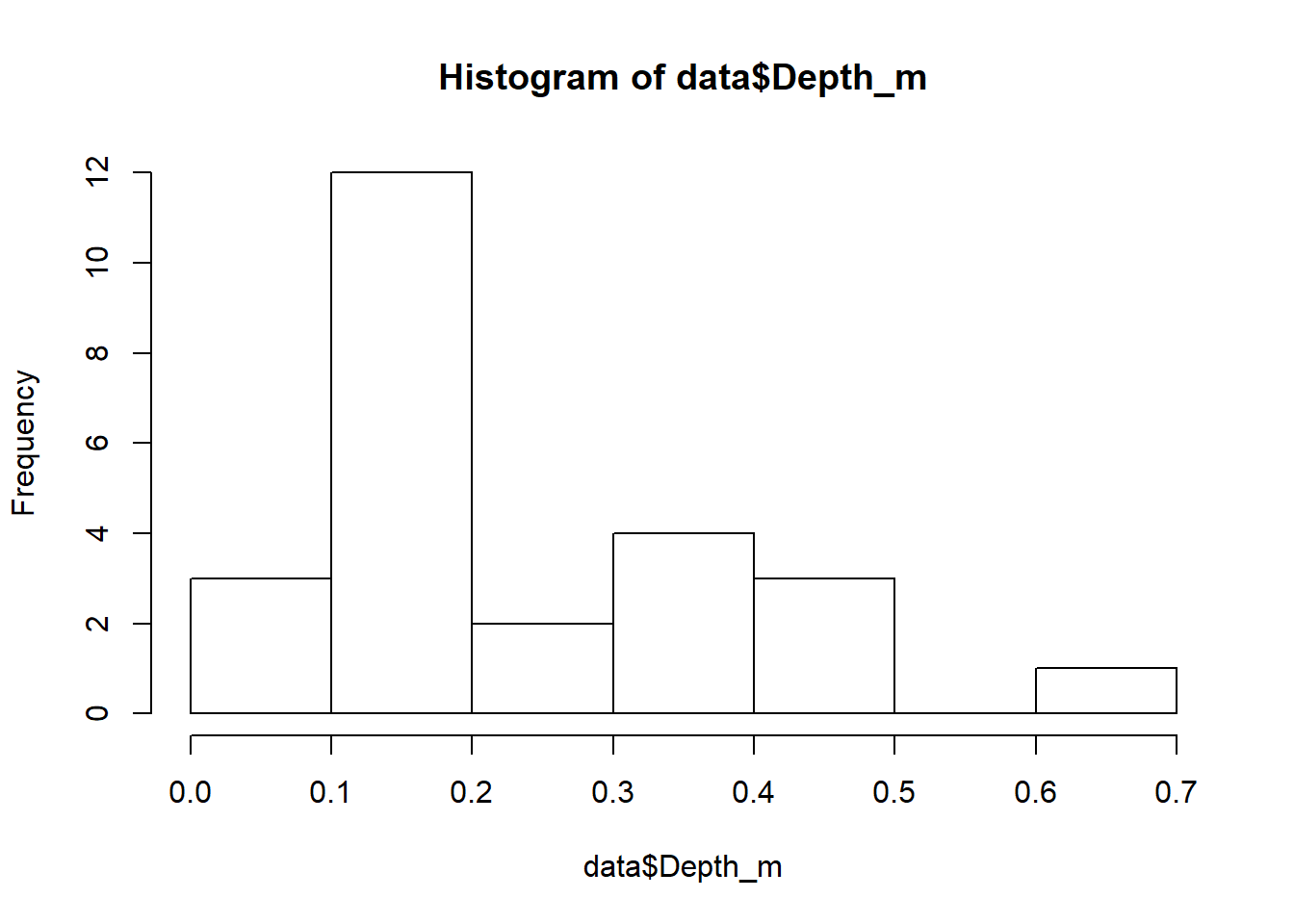Now lets make a plot of some of these parameters: # Lets see depth as we move downstream plot(data$Meters.Downstream, data$Depth_m)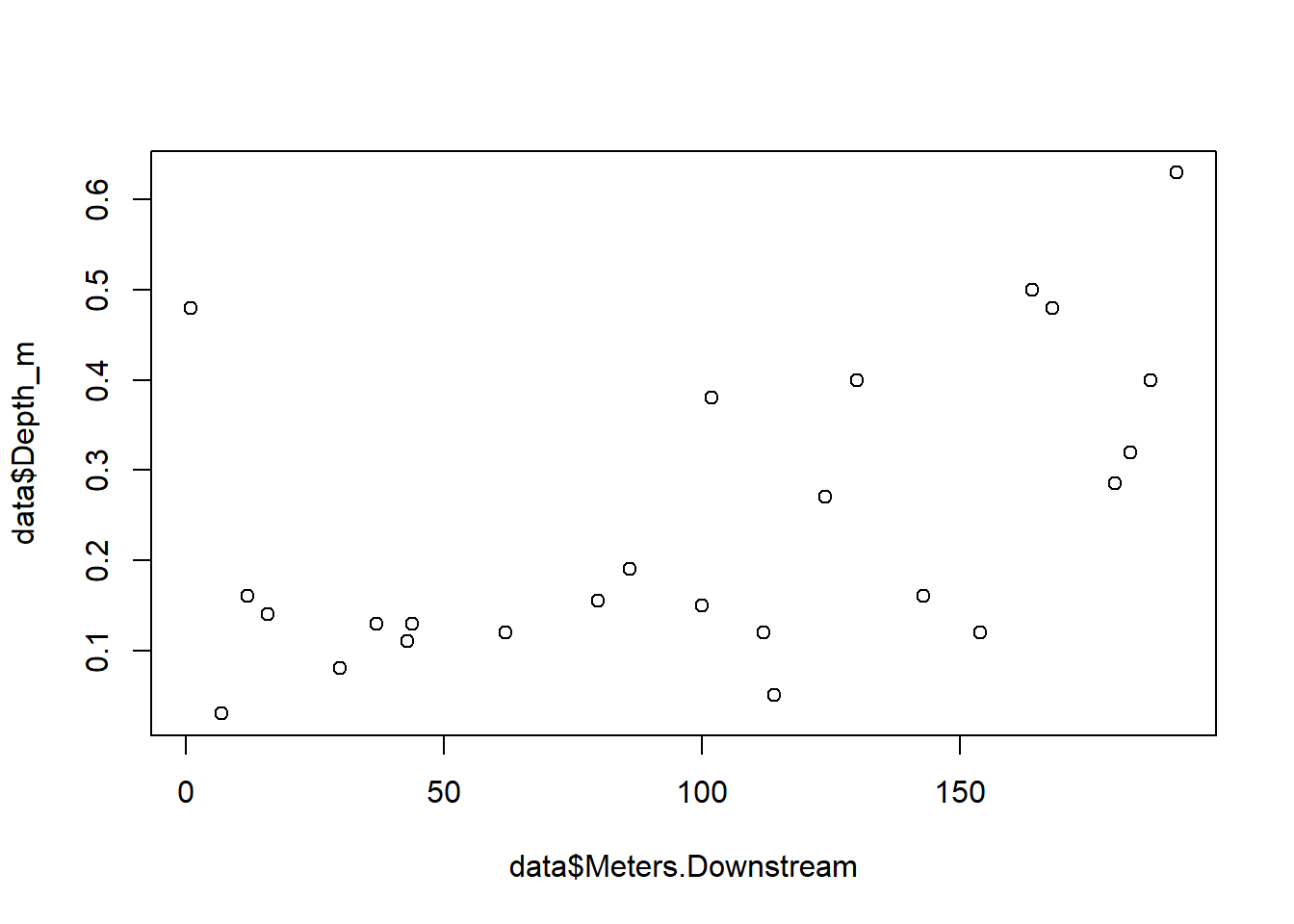Looks like it was getting deeper as the investigators moved downstream. Let’s keep that in mind for later because it might impact the relationships we observe in the data. But first, let’s jazz this plot up a bit… plot(data$Meters.Downstream, data$Depth_m, xlab = "Distance downstream (meters)", #add a nice axis labels ylab = "Depth (meters)", #and one for the y axis pch = 24, #change the symbol cex = 2, #change symbol siz main = "Depth across Eno River sample sites", #add a nice title col = "blue", #choose a color bg = "aquamarine3" #and symbol fill color )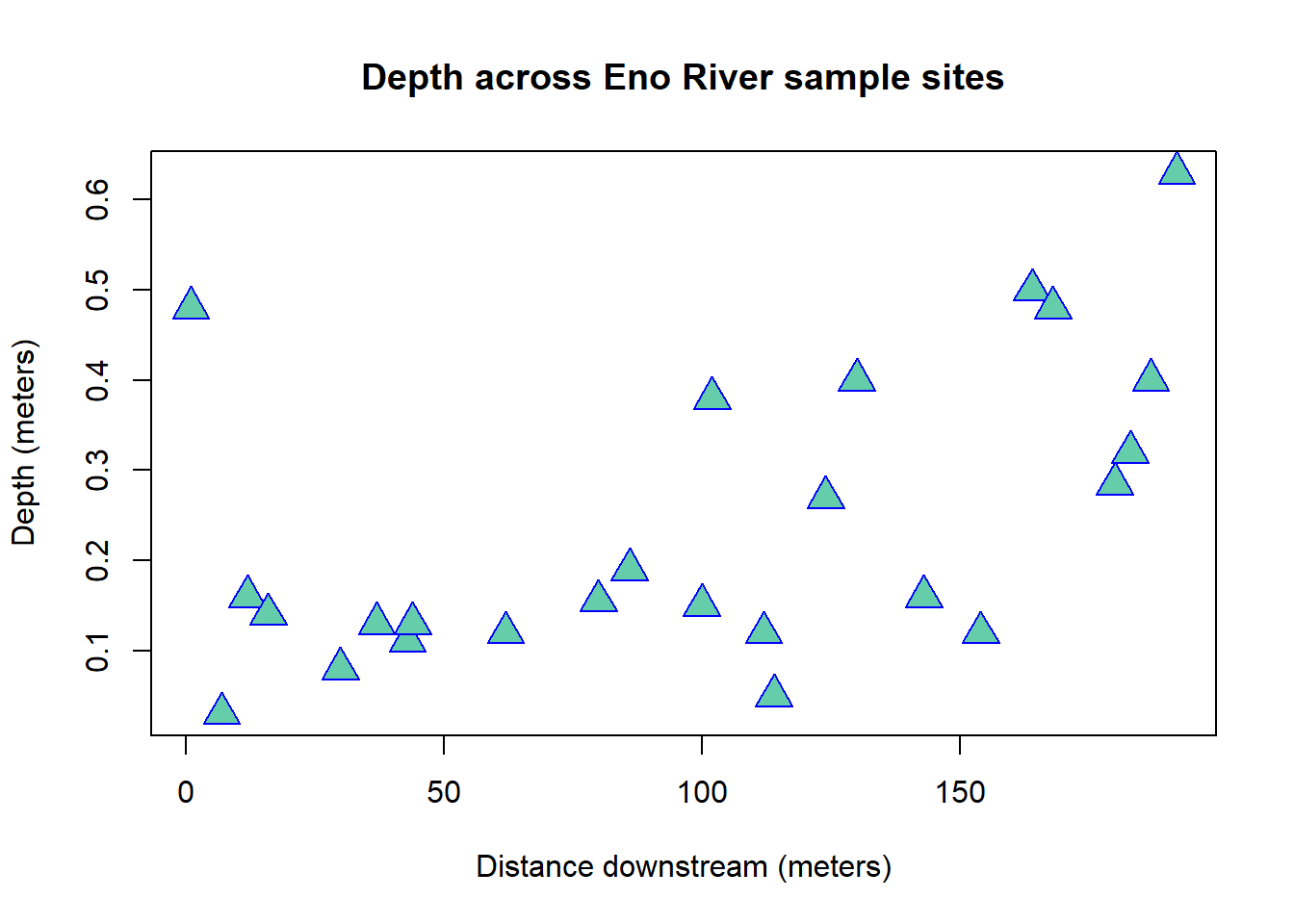Don’t get to carried away with colors, good plots keep the use of color simple, but for reference check out the website: https://www.nceas.ucsb.edu/~frazier/RSpatialGuides/colorPaletteCheatsheet.pdf and some basic graphical parameter info: https://www.statmethods.net/advgraphs/parameters.html Ok, what about some variables that we think might be correlated? Pick two and create your own plot here: # we chose temperature and pH plot(data$temperature_C, data$pH, xlab = "Temperature (degrees C", ylab = "pH", main = "Temperature versus pH at the Eno River", pch = 17, col = 4)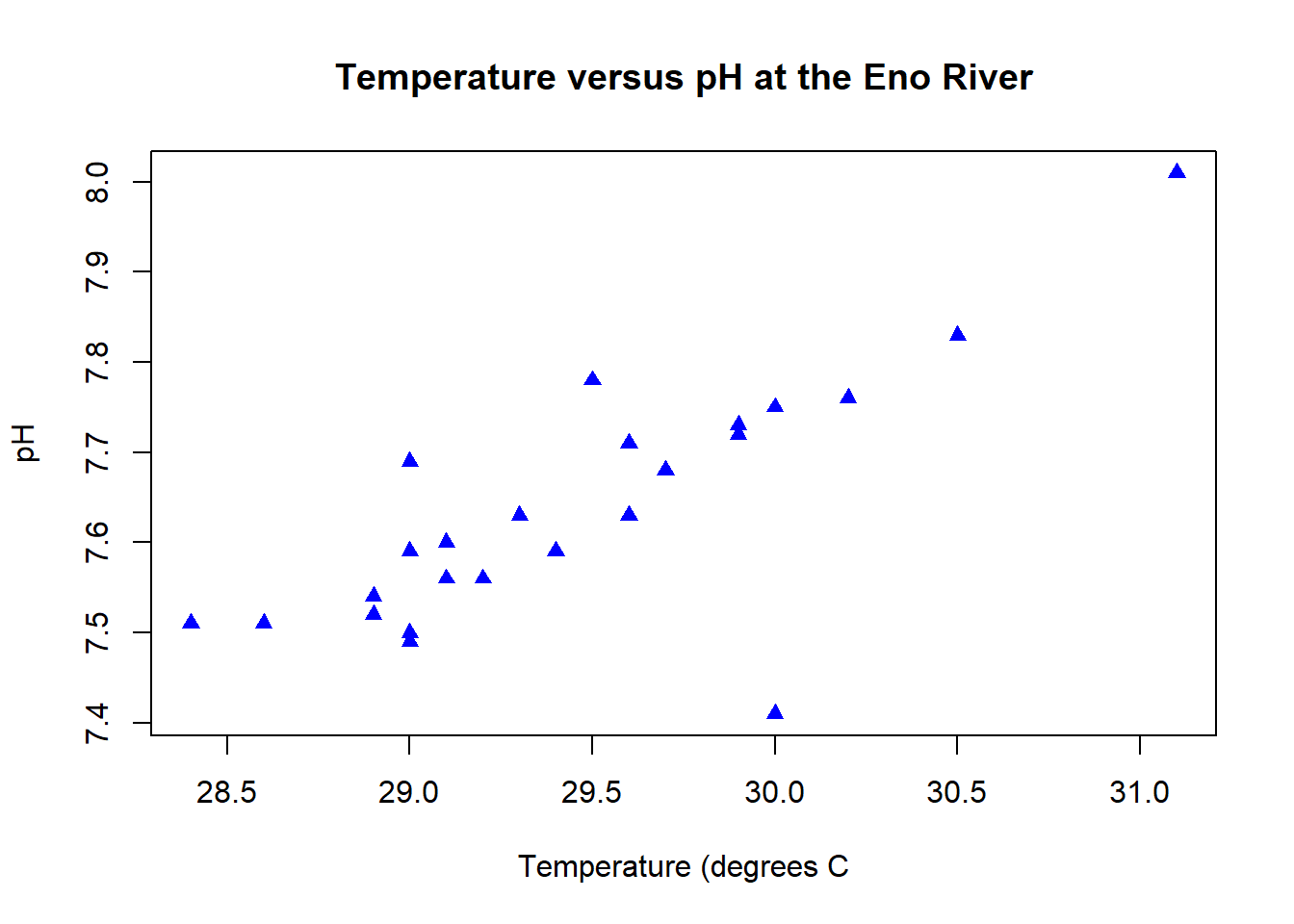Dang! That looks like a stong correlation. But how strong? We can use a linear regression model to check the strength of this relationship. fit <- glm(pH~temperature_C, data=data) #store the model output as "fit" fit #now look at the fit ## ## Call: glm(formula = pH ~ temperature_C, data = data) ## ## Coefficients: ## (Intercept) temperature_C ## 2.7381 0.1663 ## ## Degrees of Freedom: 24 Total (i.e. Null); 23 Residual ## Null Deviance: 0.421 ## Residual Deviance: 0.1682 AIC: -48.09 summary(fit) #and get summary statistics of the model ## ## Call: ## glm(formula = pH ~ temperature_C, data = data) ## ## Deviance Residuals: ## Min 1Q Median 3Q Max ## -0.31686 -0.03034 0.00977 0.02314 0.13629 ## ## Coefficients: ## Estimate Std. Error t value Pr(>|t|) ## (Intercept) 2.73815 0.83303 3.287 0.00323 ** ## temperature_C 0.16629 0.02829 5.879 5.43e-06 *** ## --- ## Signif. codes: 0 '***' 0.001 '**' 0.01 '*' 0.05 '.' 0.1 ' ' 1 ## ## (Dispersion parameter for gaussian family taken to be 0.007314263) ## ## Null deviance: 0.42102 on 24 degrees of freedom ## Residual deviance: 0.16823 on 23 degrees of freedom ## AIC: -48.086 ## ## Number of Fisher Scoring iterations: 2 Yep, it looks like the relationship is significant (see how small that p-value is, definitely less than 0.05) And the R-squared value is 0.6 indicating that the model explains about 60% of the variation in the observations. For ecology data, that’s pretty good! Want to plot the fit?! plot(data$temperature_C, data$pH, xlab = "Temperature (degrees C", ylab = "pH", main = "Temperature versus pH at the Eno River", pch = 17, col = 4) abline(fit) #adds the line to the plot text(28.75,8, "pH = 0.1663*(temp) + 2.7381", cex = 0.8, font = 3) text(28.75,7.96,paste("R^2 = ", format(summary(fit)$r.squared, digits=4)), cex = 0.8)

text(28.75,7.92,paste("p = ", format(summary(fit)$coefficients[2,4], digits=4)), cex = 0.8)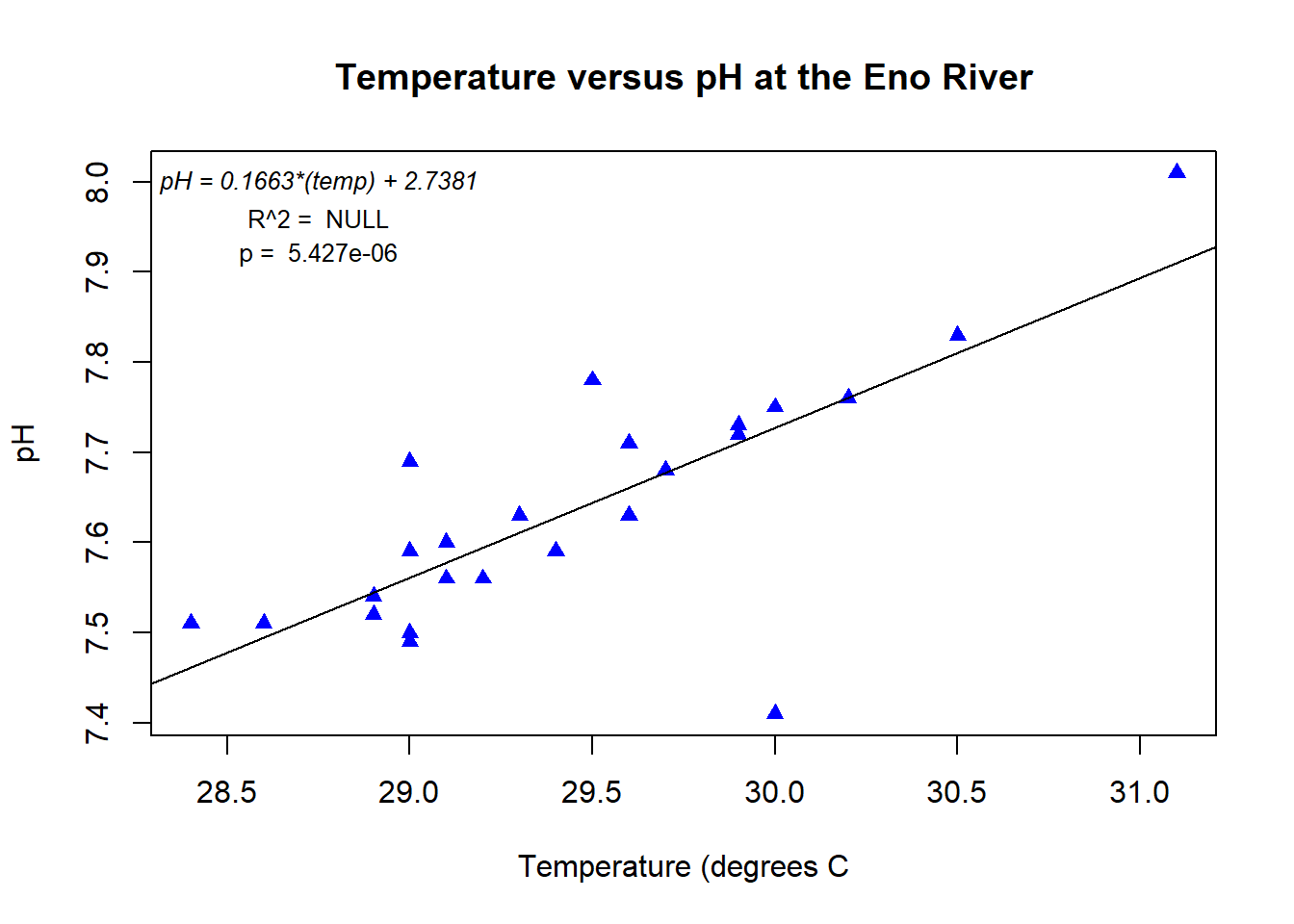# Now you try – plot a few more relationships between variables. Do you find any other significant relationships? (Use control-alt-I to insert a new code chunk) ## your code here: Ready to take it to the next level? Let’s do a linear model with more than one explanitory variable First, lets see if velocity predicts stream oxygen concentration plot(data$Velocity, data$DO_mgL)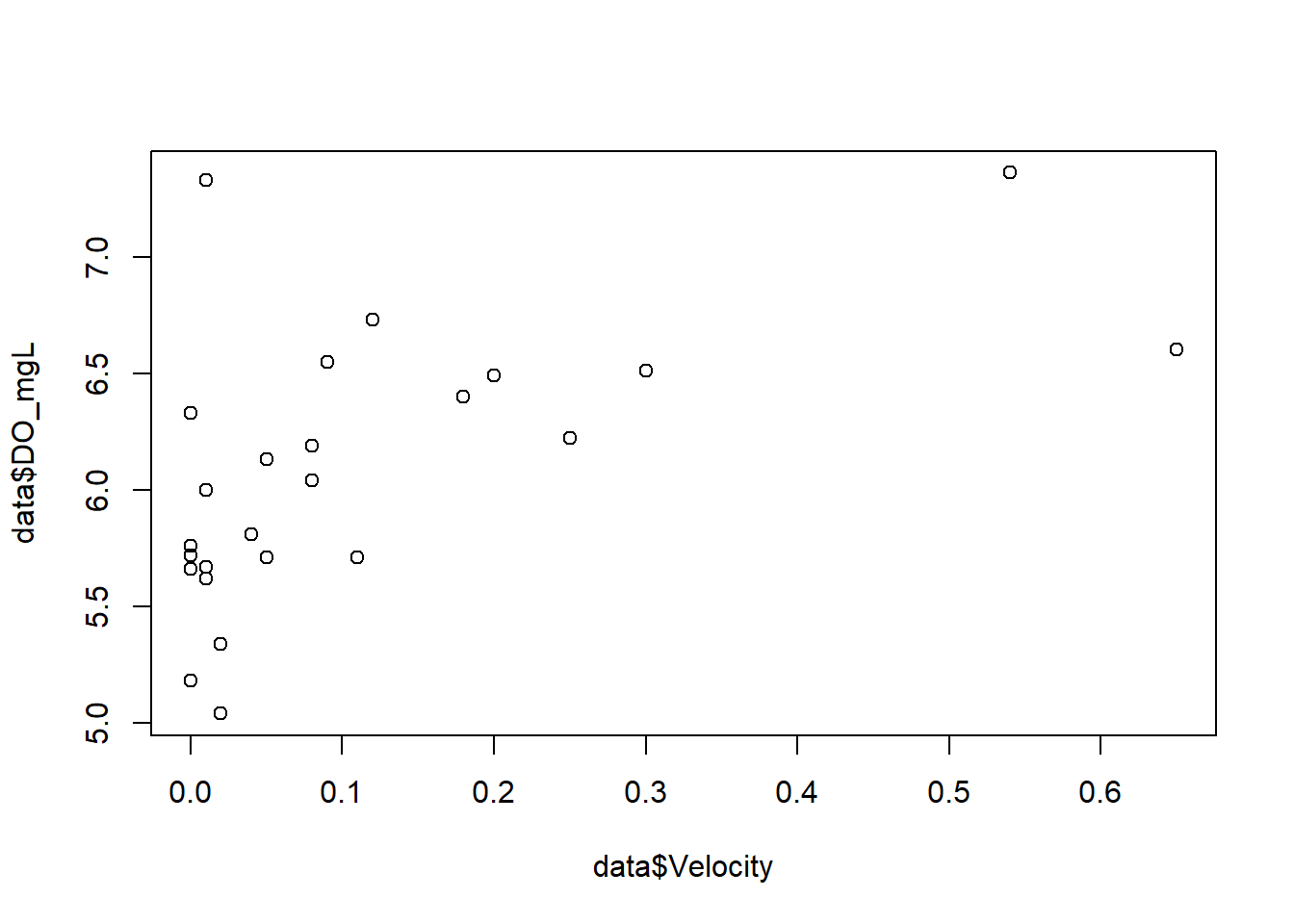DO.model1 <- lm(DO_mgL ~ Velocity, data = data) summary(DO.model1) ## ## Call: ## lm(formula = DO_mgL ~ Velocity, data = data) ## ## Residuals: ## Min 1Q Median 3Q Max ## -0.85907 -0.24885 -0.09922 0.18209 1.45086 ## ## Coefficients: ## Estimate Std. Error t value Pr(>|t|) ## (Intercept) 5.8592 0.1193 49.121 <2e-16 *** ## Velocity 1.9928 0.5981 3.332 0.0029 ** ## --- ## Signif. codes: 0 '***' 0.001 '**' 0.01 '*' 0.05 '.' 0.1 ' ' 1 ## ## Residual standard error: 0.4918 on 23 degrees of freedom ## Multiple R-squared: 0.3255, Adjusted R-squared: 0.2962 ## F-statistic: 11.1 on 1 and 23 DF, p-value: 0.002901 There appears to be a relationship, though it is not strong. Perhaps adding temperature will stregthen the model: DO.model2 <- glm(DO_mgL ~ Velocity + temperature_C, data = data) summary(DO.model2) # look at the output ## ## Call: ## glm(formula = DO_mgL ~ Velocity + temperature_C, data = data) ## ## Deviance Residuals: ## Min 1Q Median 3Q Max ## -0.74855 -0.40687 0.08191 0.27314 0.85677 ## ## Coefficients: ## Estimate Std. Error t value Pr(>|t|) ## (Intercept) -5.1171 4.3843 -1.167 0.255646 ## Velocity 2.2057 0.5462 4.039 0.000549 *** ## temperature_C 0.3720 0.1485 2.504 0.020176 * ## --- ## Signif. codes: 0 '***' 0.001 '**' 0.01 '*' 0.05 '.' 0.1 ' ' 1 ## ## (Dispersion parameter for gaussian family taken to be 0.1967873) ## ## Null deviance: 8.2484 on 24 degrees of freedom ## Residual deviance: 4.3293 on 22 degrees of freedom ## AIC: 35.11 ## ## Number of Fisher Scoring iterations: 2 Yes! We have improved the R-squared value by adding a second predictor variable to the model. FYI: the model output gives you two R squared values, the multiple R-squared and adjusted R-squared. The difference between these two is the multiple R-squared tells you the total amount of variation of your dependent variable is explained by your predictors. The adjustedR-squared is a reduced value that accounts for the use of more predictor variables to help avoid overfitting. So from here on we will refer to the adjusted R-squared value when evaluating our models. Now let’s use our model to predict oxygen level at the sample sites. # add a list of predicted values to the data frame and sort the data by velocity so that we can plot the predictions data$DOpreds<- DO.model2$fitted.values sort.data <- data[order(data$Velocity),]

plot(data$Velocity, data$DO_mgL, xlab = "Velocity", ylab = "DO (mg/L)")

# add the predictions from linear model as a dashed line

lines(sort.data$Velocity, sort.data$DOpreds, lty = 2)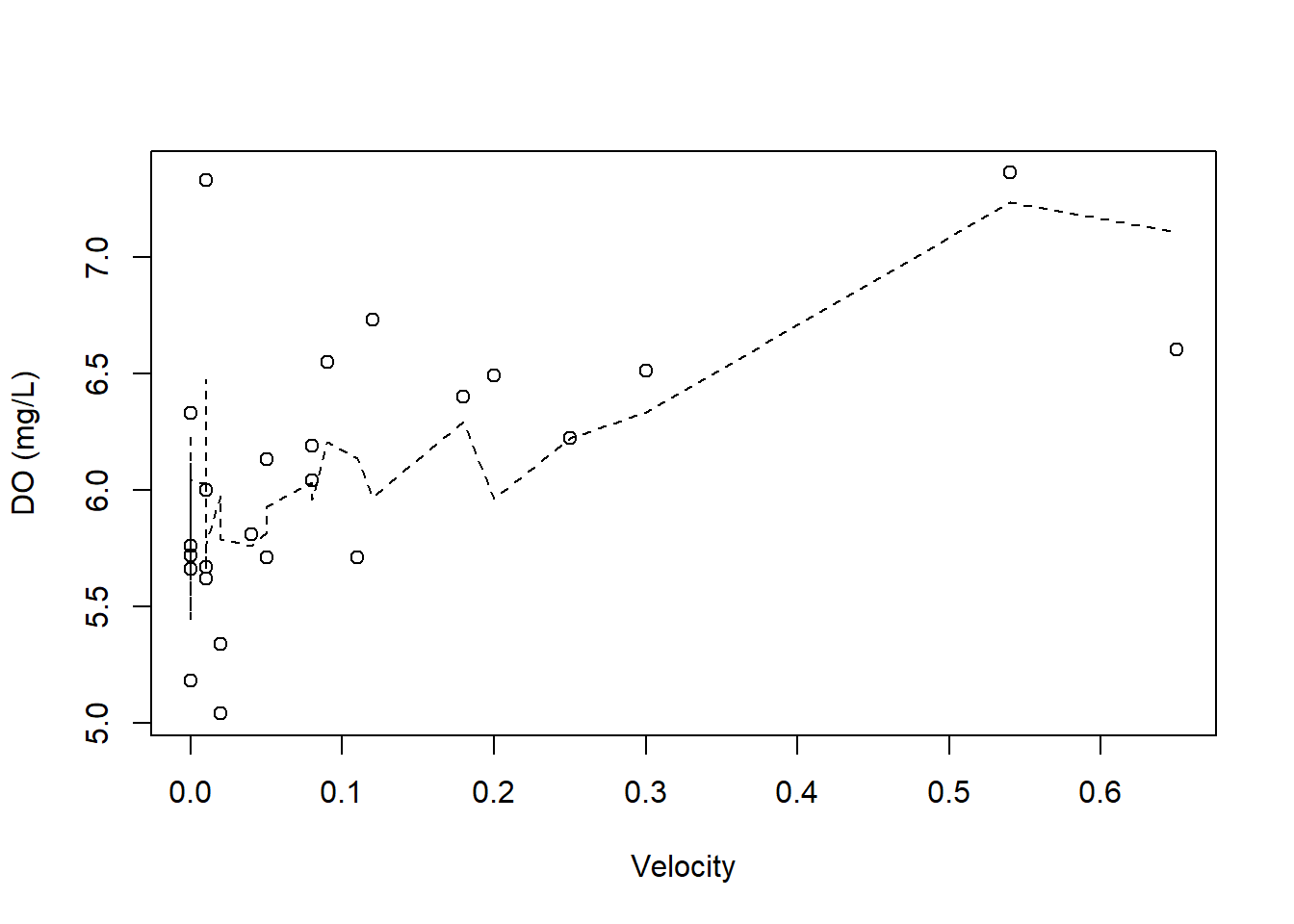# Now you try:

Often ecological data is not linear, particularly concentration data (DO, conductivity) What happens if you try to transform the data, does this improve the model fit more than adding Temperature as a second predictor? Try a log transformation on the data:

# your code here

## Binning Continuous Data

Can you predict how many snails will be found given the physical parameters of a site? What varibale might affect where snails live? Build your own model with one or more variables

Not sure where to start? Try making a box plot with some of your variables

boxplot(data$snail_count, ylab = "snail count", xlab = "all sites")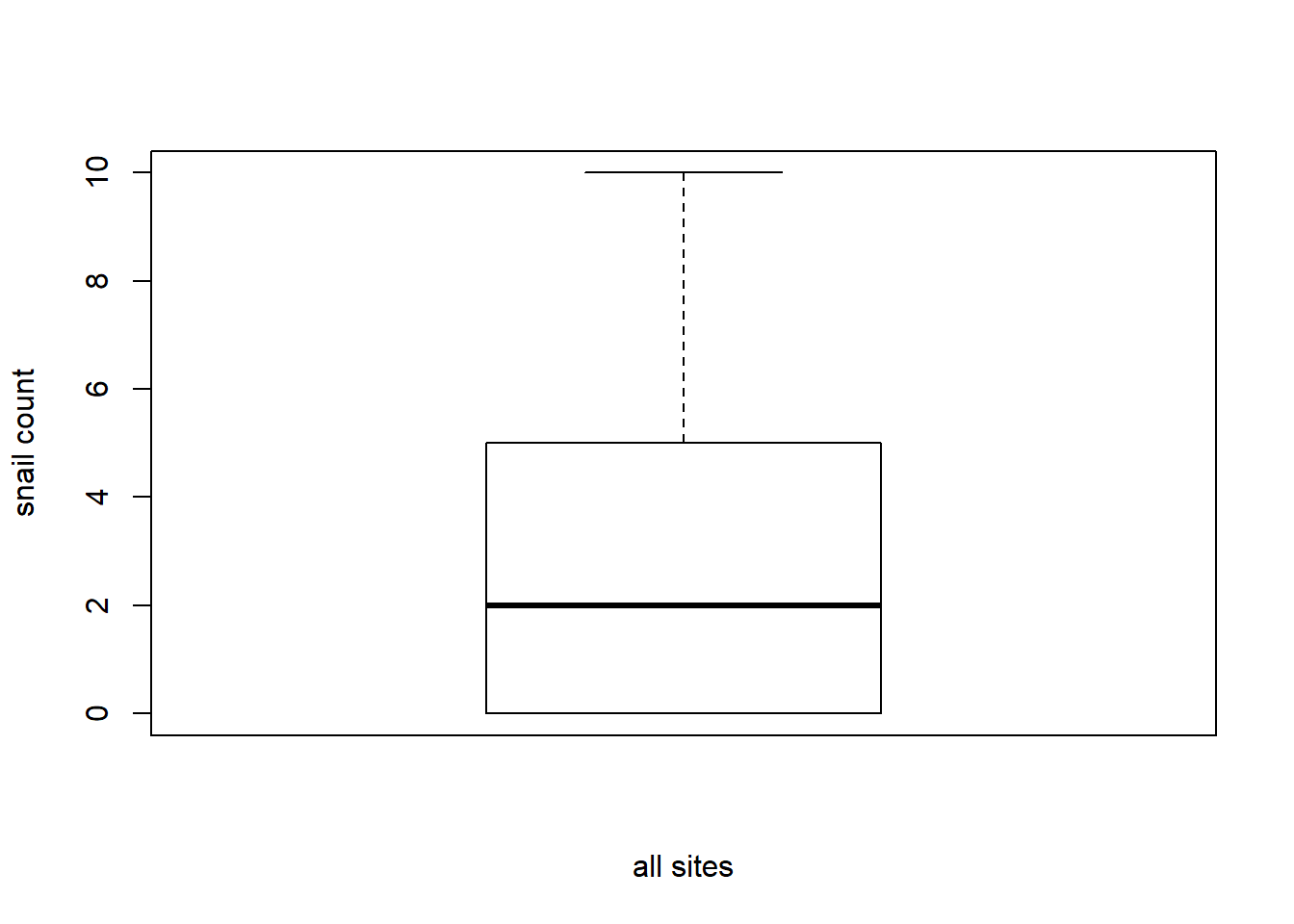# you can look at the distribution of snails across binned values of another variable: # Create 4 bins of the DO data, with the same number of points in each bin breaks <- quantile(data$DO_mgL)                 # this creates a vector of the s

data$DO_bins <- cut(data$DO_mgL, breaks)        # add a column of the DO bins

boxplot(data$snail_count~data$DO_bins, xlab = "Dissolved oxygen (mg/L)",

ylab = "Snail count", main = "Snail distribution by DO")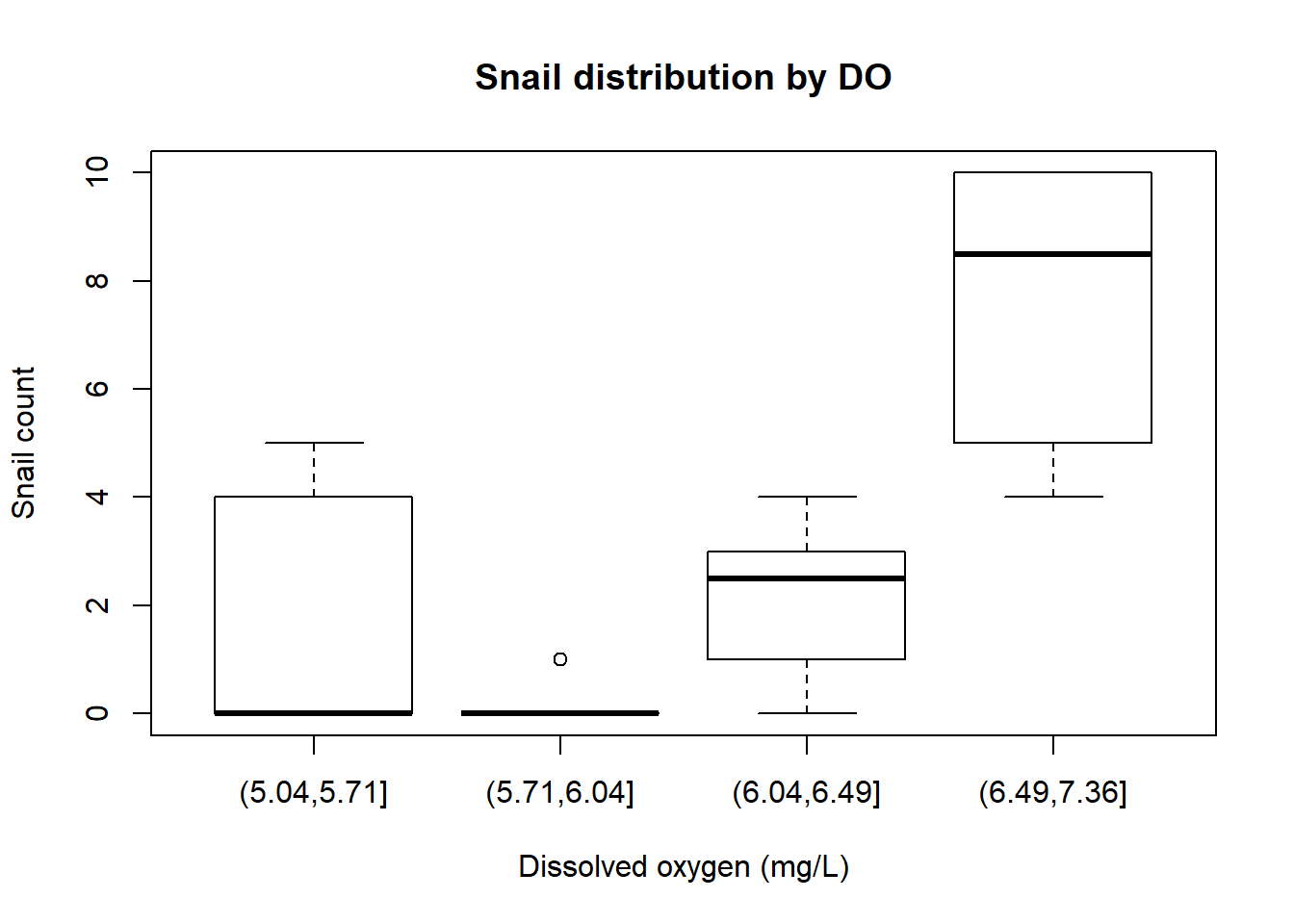## fix the x axis by muting the default: xaxt="n"

breaks <- quantile(data$DO_mgL) # this creates a vector of the s data$DO_bins <- cut(data$DO_mgL, breaks) # add a column of the DO bins boxplot(data$snail_count~data$DO_bins, xlab = "Dissolved oxygen (mg/L)", ylab = "Snail count", main = "Snail distribution by DO", xaxt="n") ## and writing your own axis(1, at = 1:4, labels= c("5.04-5.71", "5.71-6.04", "6.04-6.49", "6.49-7.36"))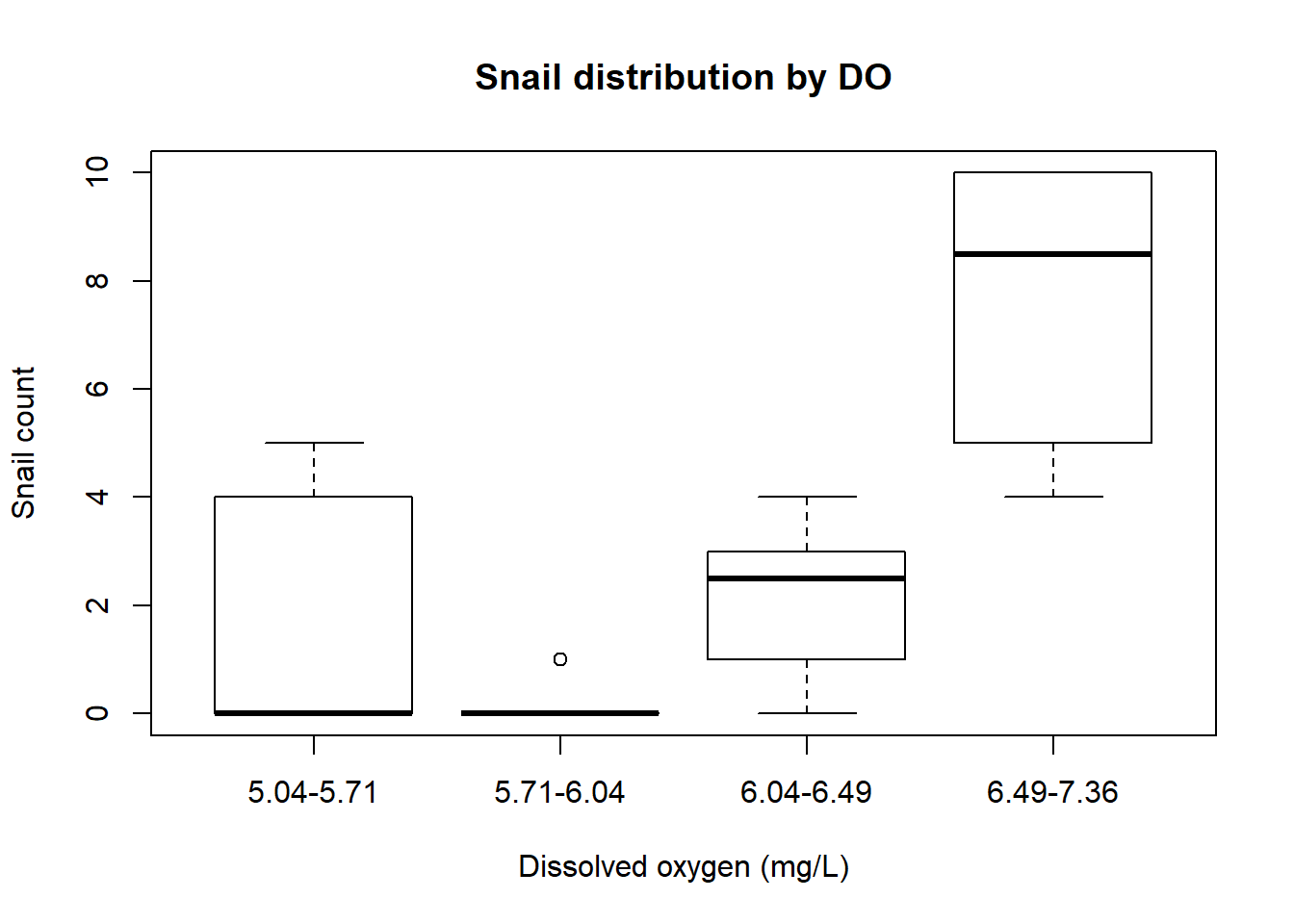You can recreate these bins for wahtever variable and intervals you want by creating a vector called breaks by entering numbers like this: breaks <- c(5,6,7,8) data$DO_bins <- cut(data$DO_mgL, breaks) # add a column of the DO bins boxplot(data$snail_count~data$DO_bins, xlab = "Dissolved oxygen level", ylab = "Snail count", main = "Snail distribution by DO", xaxt="n", col = c("lightblue1", "lightblue3", "lightblue4")) axis(1, at = 1:3, labels= c("low", "medium", "high")) legend("bottomright", inset = 0.02, title = "DO (mg/L)", c("5-6", "6-7", "7-8"), fill = c("lightblue1", "lightblue3", "lightblue4"), cex = 0.8)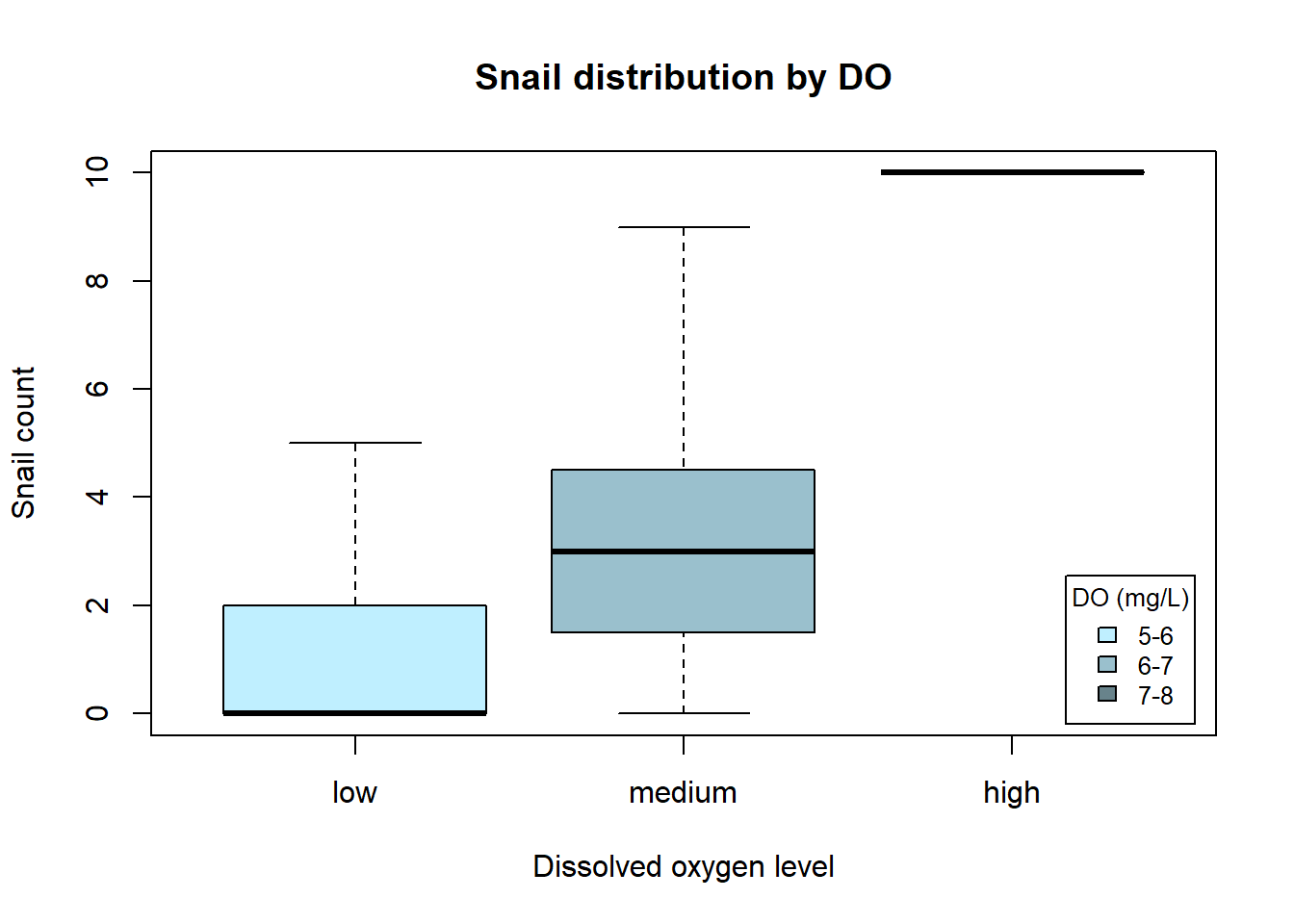## Dealing with Count data We can see that this data doesn’t look linear, and we know that it isn’t continuous because you can’t have 0.5 snails! So let’s try modeling our data with a poisson distribution, which is specifically designed for this type of data. model <- glm(data$snail_count~data$DO_mgL, family = poisson()) summary(model) ## ## Call: ## glm(formula = data$snail_count ~ data$DO_mgL, family = poisson()) ## ## Deviance Residuals: ## Min 1Q Median 3Q Max ## -2.2242 -1.5851 -0.4120 0.5683 2.6113 ## ## Coefficients: ## Estimate Std. Error z value Pr(>|z|) ## (Intercept) -7.1597 1.2504 -5.726 1.03e-08 *** ## data$DO_mgL   1.3030     0.1897   6.868 6.49e-12 ***

## ---

## Signif. codes:  0 '***' 0.001 '**' 0.01 '*' 0.05 '.' 0.1 ' ' 1

##

## (Dispersion parameter for poisson family taken to be 1)

##

##     Null deviance: 101.485  on 24  degrees of freedom

## Residual deviance:  54.833  on 23  degrees of freedom

## AIC: 107.7

##

## Number of Fisher Scoring iterations: 6

We see this is giving us significant results! To be sure this is the model we should be using, we really should check that the data are poisson distributed. How about you give that a try?

# your code here

Now that we are convinced, lets see what the poisson model fit looks like in a plot:

plot(data$DO_mgL, data$snail_count,

xlab = "DO (mg/L)", ylab = "snail count",

pch = 20, col = "grey40")

b0 <-  model$coef # intercept b1 <- model$coef           # slope

SE0 <-  sqrt(diag(vcov(model))) #standard error for the intercept

SE1 <-  sqrt(diag(vcov(model)))# standard error for the slope

# create a sequence of x values that cover the range of DO values

x<-seq(min(data$DO_mgL), max(data$DO_mgL), length.out = nrow(data))

# generate model predicted snail counts

y <- exp(b0+b1*x)

ylower <- exp((b0-SE0)+(b1-SE1)*x)

yupper <- exp((b0+SE0)+(b1+SE1)*x)

lines(x, y)

lines(x,ylower, lty = 2)

lines(x,yupper, lty=2)